# Identify the Area of a Rectangle

In this worksheet students will learn the formula for finding the area of a rectangle and be able to find areas.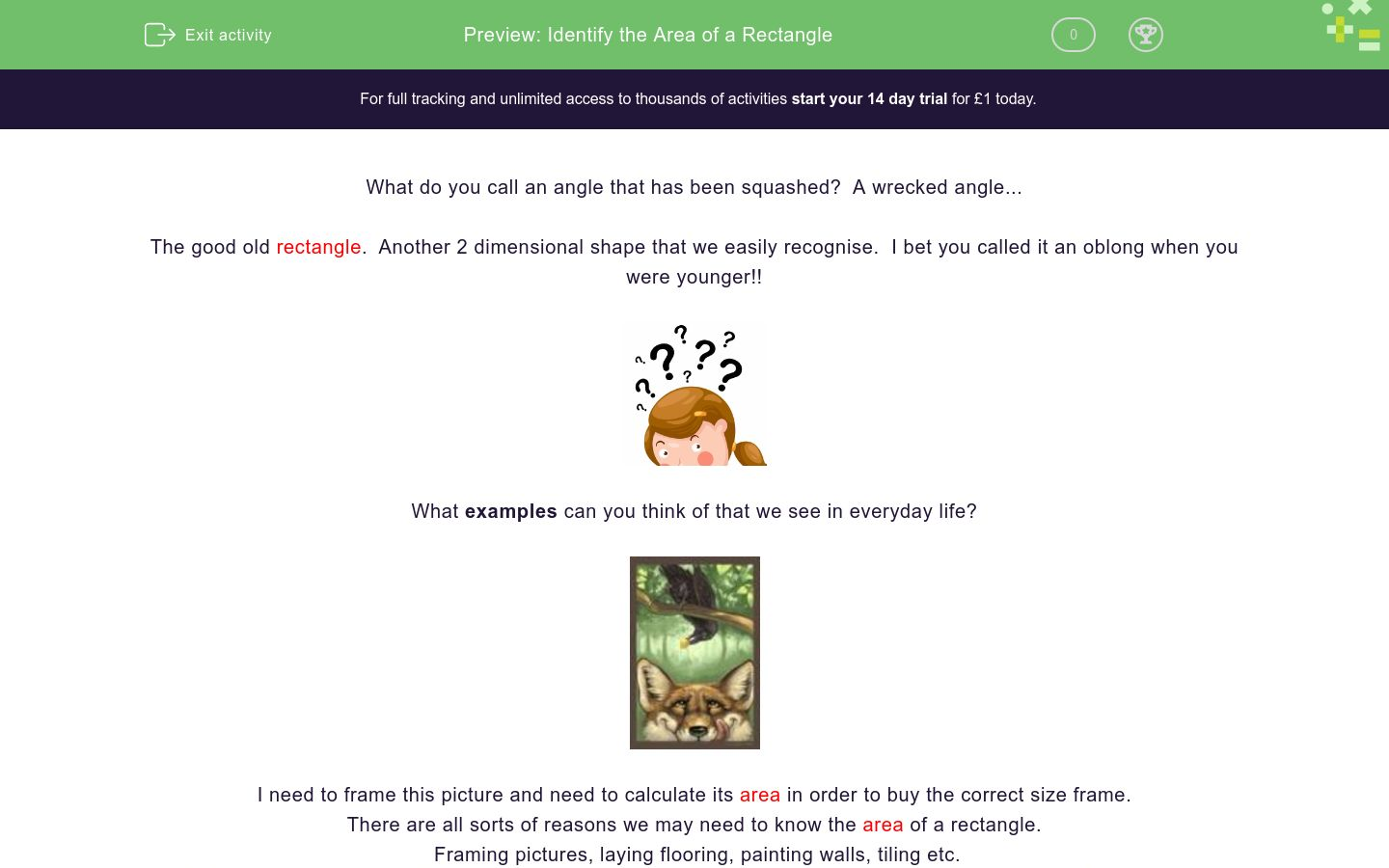Key stage:  KS 4

GCSE Subjects:   Maths

GCSE Boards:   AQA, Eduqas, Pearson Edexcel, OCR

Curriculum topic:   Geometry and Measures, Mensuration

Curriculum subtopic:   Mensuration and Calculation, Area Calculations

Difficulty level:### QUESTION 1 of 10

What do you call an angle that has been squashed?  A wrecked angle...

The good old rectangle.  Another 2 dimensional shape that we easily recognise.  I bet you called it an oblong when you were younger!!What examples can you think of that we see in everyday life?I need to frame this picture and need to calculate its area in order to buy the correct size frame.

There are all sorts of reasons we may need to know the area of a rectangle.

Framing pictures, laying flooring, painting walls, tiling etc.

Before we can do any of this there is one thing we need to know and that is how to find the area of a rectangle.

First of all, we know that the sides of a rectangle are not all the same length.

Two sides will have one length and the other two sides a different length.As with a lot of shape work there is a formula that you need to learn. So here goes:

Area of a rectangle base x height

Yes, really that is all there is to it.

You may recognise that it is the same as the formula for the area of a square.

Find the area of this rectangle:Area = base x height

Area = 9 cm x 5 cm = 45 cm² As we are working with area units are always squared. e.g cm² m²

There will be no stopping you now!Find the area of this rectangle. The lengths are 6 cm and 8 cm.

14 cm²

36 cm²

48 cm²

64 cm²

Can you match the description of the rectangle to the correct area?

## Column B

Rectangle sides 4 cm and 3cm
22.26 m²
Rectangle sides 6 cm and 5 cm
30 cm²
Rectangle sides 11 cm and 3 cm
20 cm²
Rectangle sides 8.5 cm and 7.3 cm
33 cm²
Rectangle sides 4cm and 5 cm
12 cm²
rectangle sides 5.3 m x and 4.2 m
62.05 cm²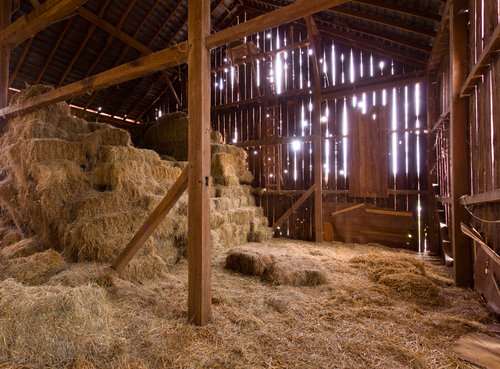A farmer has a rectangular barn for his sheep measuring  2.7m along one wall and 212 cm along the other wall.  What is the area of the floor of the barn?

57240 cm²

5.624 m²

5.724 m²

5.64 m²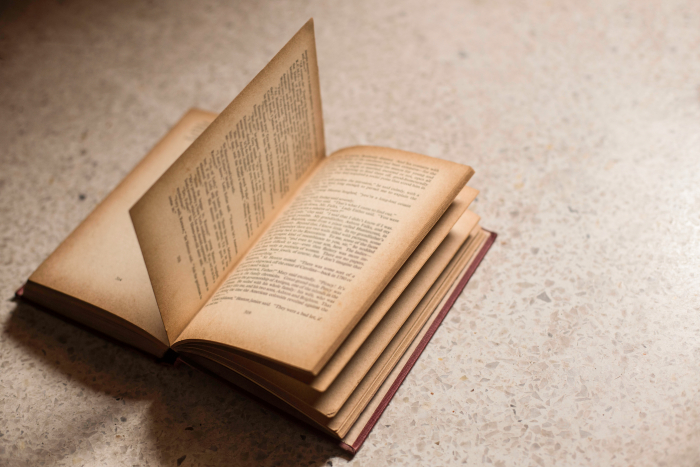Find the area of a page of this book.  The width of a page is 6 mm and the height is 8 mm.

57240 cm²

5.624 m²

5.724 m²

5.64 m²What is the difference between a rectangular cricket bat with side lengths of 45 cm and 4 cm and a bat of 35 by 5.2 cm?

57240 cm²

5.624 m²

5.724 m²

5.64 m²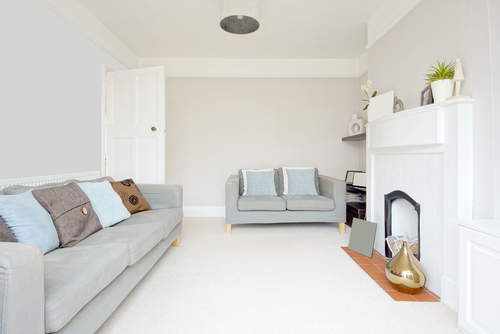An L shaped living room and dining room need a new carpet?

The living room is a rectangle of  6 m by 5 m.  The dining room is 6m by 4m.  What is the total area of carpet that is needed for the floor?

30 m²

54 m²

64 cm²

54 cm²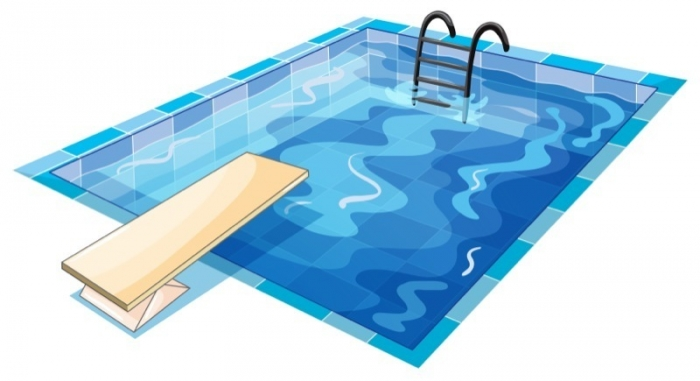A swimming pool has sides of  25 m by 6 m.  There is a border of tiles 2 m around the whole pool.

 125 m² 200 m² 150 m² 48 m² 30 m² 50 m² 70 m² What is the area of the pool? What is the area of the tiles that surround the pool?Lara is completing an abstract painting made up of rectangles to put in this picture frame.  She has painted, red rectangles, yellow rectangles, and blue rectangles.

The red rectangles have sides of 3 cm and 2 cm

The blue rectangles have sides of 4 cm and 5 cm

The green rectangles have sides of 3 cm and 7 cm

Lara has 3 blue, 2 yellow and 1 red rectangle in the picture.  What is the total area of the picture?

 125 m² 200 m² 150 m² 48 m² 30 m² 50 m² 70 m² What is the area of the pool? What is the area of the tiles that surround the pool?What is the area of this rectangle with sides x and x + 3

x times x + 3

x² +3

4x + 6

x² +3xWhat is the  area of this rectangle with sides 7 cm and x + 4.

11x + 4

7x + 28

x + 4 x 7

2x +22

• Question 1Find the area of this rectangle. The lengths are 6 cm and 8 cm.

48 cm²
EDDIE SAYS
Did you apply the formula? Base x height When working with an area of a rectangle you are usually given both side lengths. 6 x 8= 48 cm²
• Question 2

Can you match the description of the rectangle to the correct area?

## Column B

Rectangle sides 4 cm and 3cm
12 cm²
Rectangle sides 6 cm and 5 cm
30 cm²
Rectangle sides 11 cm and 3 cm
33 cm²
Rectangle sides 8.5 cm and 7.3 cm
62.05 cm²
Rectangle sides 4cm and 5 cm
20 cm²
rectangle sides 5.3 m x and 4.2 m
22.26 m²
EDDIE SAYS
Applying the formula is now getting easy. Make sure you read both numbers carefully 4 x 3 = 12 cm² 5 x 6 = 30 cm² 11 x 3 = 33 cm² 8 .5 x 7.3 = 62.05 cm² 4 x 5 = 20 cm² 5.2 x 4.2 = 22.26 cm² You're usually asked to round to 1 or 2 decimal places.
• Question 3A farmer has a rectangular barn for his sheep measuring  2.7m along one wall and 212 cm along the other wall.  What is the area of the floor of the barn?

57240 cm²
5.724 m²
EDDIE SAYS
Often area questions will give two different units of measurement. You will need your eagle eye for this. You can convert either way: 2.7 m = 270 cm 270 x 212 =57,240 cm² or 212 cm = 2.12 m 2.7 x 2.12 =5.724 (5.73 to 3 d.p) m² They can't pull the wool over your eyes!
• Question 4Find the area of a page of this book.  The width of a page is 6 mm and the height is 8 mm.

EDDIE SAYS
See you can apply the formula to anything that is rectangular in shape. 6 x 8 = 48 mm² Providing you select the correct numbers you will never go wrong.
• Question 5What is the difference between a rectangular cricket bat with side lengths of 45 cm and 4 cm and a bat of 35 by 5.2 cm?

EDDIE SAYS
This is just another example of finding area from a written question. 45 x 4 = 180 cm² 35 x 5.2 cm = 182² 182 - 180 = 2cm² The word difference in a question should lead you to subtract. Howzat!!
• Question 6An L shaped living room and dining room need a new carpet?

The living room is a rectangle of  6 m by 5 m.  The dining room is 6m by 4m.  What is the total area of carpet that is needed for the floor?

54 m²
EDDIE SAYS
This is just a simple 2 step question. You have all the knowledge that you need. Area of living room = 6 x 5 = 30 m² Area of dining room = 6 x 4 = 24 m² Add the two together: 30 + 24 = 54 m² You could charge people for working out stuff like this.
• Question 7A swimming pool has sides of  25 m by 6 m.  There is a border of tiles 2 m around the whole pool.

 125 m² 200 m² 150 m² 48 m² 30 m² 50 m² 70 m² What is the area of the pool? What is the area of the tiles that surround the pool?
EDDIE SAYS
This is a problem tilers face all the time when part of an area needs tiling. The first part is second nature to you now. Swimming pool 25 x 6 = 150 m² The next part takes a little more thought. Find the total area of the pool a border. 25 x 8 = 200 m² Subtract this from the area of the pool, to leave you with the tiled area: 200 - 150 = 50 m² This is no problem for you diving into a question like this.
• Question 8Lara is completing an abstract painting made up of rectangles to put in this picture frame.  She has painted, red rectangles, yellow rectangles, and blue rectangles.

The red rectangles have sides of 3 cm and 2 cm

The blue rectangles have sides of 4 cm and 5 cm

The green rectangles have sides of 3 cm and 7 cm

Lara has 3 blue, 2 yellow and 1 red rectangle in the picture.  What is the total area of the picture?

EDDIE SAYS
Lets not gloss over this! Find the area of each coloured rectangle. Multiply it up by the number required for the picture Add them all together. Blue 4 x 5 = 20 x 3 = 60 Yellow 3 x 7 = 21 x 2 = 42 Red 3 x 2 = 6 60 + 42 + 6 = 108 cm²
• Question 9What is the area of this rectangle with sides x and x + 3

x times x + 3
x² +3x
EDDIE SAYS
I know what you're thinking...eeekkk! This is easier than it looks. We know that 1 side is length x The other side is length x + 3. We don't know what x is so all we can write is: x multiplied by x + 3 or if you want to show of x times x = x² x times 3 = 3x which is x² + 3x
• Question 10What is the  area of this rectangle with sides 7 cm and x + 4.

7x + 28
x + 4 x 7
EDDIE SAYS
Bring it on!! 7 multiplied by x + 4. (one side multiplied by the other) or showing off again 7x + 28. If you don't know how I got 7x + 28. Look at multiplying out brackets.
---- OR ----

Sign up for a £1 trial so you can track and measure your child's progress on this activity.

### What is EdPlace?

We're your National Curriculum aligned online education content provider helping each child succeed in English, maths and science from year 1 to GCSE. With an EdPlace account you’ll be able to track and measure progress, helping each child achieve their best. We build confidence and attainment by personalising each child’s learning at a level that suits them.

Get started